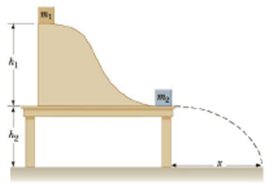Chapter 6, Problem 71AP

Chapter
Section
Textbook Problem

A block with mass m1 = 0.500 kg is released from rest on a frictionless track at a distance h1, = 2.50 m above the top of a table. It then collides elastically with an object having mass m2 = 1.00 kg that is initially at rest on the table, as shown in Figure P6.71. (a) Determine the velocities of the two objects just after the collision. (b) How high up the track does the 0.500-kg object travel back after the collision? (c) How far away from the bottom of the table does the1.00-kg object land, given that the height of the table h2 = 2.00 m? (d) How far away from the bottom of the table does the 0.500-kg object eventually band?Figure P6.71

(a)

To determine
The velocity of each object just after the collision.

Explanation

Given info:

The acceleration due to gravity is 9.80m/s2 .

The height of the track is 2.50m .

The first mass is 0.500kg .

The mas of the second mass is 1.00kg .

Explanation:

The Formula to calculate the conservation of mechanical energy,

12m1v1i2+mg(0)=12m1(0)+m1gh1 (I)

• h1 is the height of the track
• m1 is the first mass
• g is the acceleration due to gravity
• v1i is the speed of first object

Rearranging the formula gives the speed as,

v1i=2gh1

Substitute 9.80m/s2 for g and 2.50m for h1 in equation (I) to find v1i .

v1i=2(9.80m/s2)(2.50m)=7.00m/s

The Formula to calculate the conservation of momentum just before to the collision to just after the collision,

m1v1f+m2v2f=m1v1i+0 (II)

• v1f is the speed of first bock just after collision
• v2f is the speed of second bock just after collision

Substitute 0.500kg for m1 , 1.00kg for m2 and 7.00m/s for v1i in equation (II)

(b)

To determine
The height at which the 0.500 kg object travel back after the collision.

(c)

To determine
The distance at which the 1.00 kg object lands.

(d)

To determine
The distance at which the 0.500 kg object lands.

Still sussing out bartleby?

Check out a sample textbook solution.

See a sample solution

The Solution to Your Study Problems

Bartleby provides explanations to thousands of textbook problems written by our experts, many with advanced degrees!

Get Started